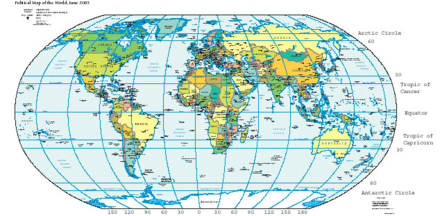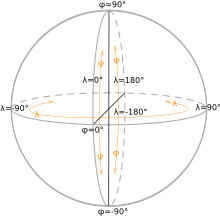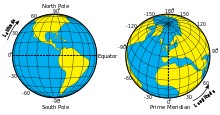# Geographic coordinate system

﻿
Geographic coordinate systemMap of Earth showing lines of latitude (horizontally) and longitude (vertically), Eckert VI projection; large version (pdf, 3.12MB)
GeodesyFundamentals
Geodesy · Geodynamics
Geomatics · Cartography
Concepts
Datum · Distance · Geoid
Figure of the Earth
Geodetic system
Geog. coord. system
Hor. pos. representation
Map projection
Reference ellipsoid
Satellite geodesy
Spatial reference system
Technologies
GNSS · GPS · ...
Standards
UTM · WGS84 · ...
History
History of geodesy
NAVD29 · ...
v · coordinate system that enables every location on the Earth to be specified by a set of numbers. The coordinates are often chosen such that one of the numbers represent vertical position, and two or three of the numbers represent horizontal position. A common choice of coordinates is latitude, longitude and elevation.

## Geographic latitude and longitudeLatitude phi (φ) and Longitude lambda (λ)Latitude and Longitude of the Earth

The geographic latitude (abbreviation: Lat., φ, or phi) of a point on the Earth's surface is the angle between the equatorial plane and a line that passes through that point and is normal to the surface of a reference ellipsoid which approximates the shape of the Earth.[n 1] This line passes a few kilometers away from the center of the Earth except at the poles and the equator where it passes through Earth's center.[n 2] Lines joining points of the same latitude trace circles on the surface of the Earth called parallels, as they are parallel to the equator and to each other. The north pole is 90° N; the south pole is 90° S. The 0° parallel of latitude is designated the equator, the fundamental plane of all geographic coordinate systems. The equator divides the globe into Northern and Southern Hemispheres.

The Longitude (abbreviation: Long., λ, or lambda) of a point on the Earth's surface is the angle east or west from a reference meridian to another meridian that passes through that point. All meridians are halves of great ellipses (often improperly called great circles), which converge at the north and south poles.

A line passing near the Royal Observatory, Greenwich (near London in the UK) has been chosen as the international zero-longitude reference line, the Prime Meridian. Places to the east are in the eastern hemisphere, and places to the west are in the western hemisphere. The antipodal meridian of Greenwich is both 180°W and 180°E. The zero/zero point is located in the Gulf of Guinea about 625 km south of Tema, Ghana.

In 1884, the United States hosted the International Meridian Conference and twenty-five nations attended. Twenty-two of them agreed to adopt the location of Greenwich as the zero-reference line. The Dominican Republic voted against the adoption of that motion, while France and Brazil abstained. To date, there exist organizations around the world which continue to use historical prime meridians which existed before the acceptance of Greenwich became common-place.[n 3]

The combination of these two components specifies the position of any location on the planet, but does not consider altitude nor depth.

This latitude/longitude "webbing" is known as the conjugate graticule.

In defining an ellipse, the short (vertical) diameter is known as the conjugate diameter, and the long (horizontal) diameter—perpendicular, or "transverse", to the conjugate—is the transverse diameter. With a sphere or ellipsoid, the conjugate diameter is known as the polar axis and the transverse as the equatorial axis. The graticule perspective is based on this designation: As the longitudinal rings — geographically defined, all great circles — converge at the poles, it is the poles that the conjugate graticule is defined. If the polar vertex is "pulled down" 90°, so that the vertex is on the equator, or transverse diameter, then it becomes the transverse graticule, upon which all spherical trigonometry is ultimately based (if the longitudinal vertex is between the poles and equator, then it is considered an oblique graticule).

1. ^ The surface of the Earth is closer to an ellipsoid than to a sphere, as its equatorial diameter is larger than its north-south diameter.
2. ^ The greatest distance between an ellipsoid normal and the center of the Earth is 21.9 km at a latitude of 45°, using Earth radius#Radius at a given geodetic latitude and Latitude#Comparison of selected types: (6367.5 km)×tan(11.67')=21.9 km.
3. ^ The French Institut Géographique National (IGN) maps still use longitude from a meridian passing through Paris, along with longitude from Greenwich.

## UTM and UPS systems

The Universal Transverse Mercator (UTM) and Universal Polar Stereographic (UPS) coordinate systems both use a metric-based cartesian grid laid out on a conformally projected surface to locate positions on the surface of the Earth. The UTM system is not a single map projection but a series of map projections, one for each of sixty 6-degree bands of longitude. The UPS system is used for the polar regions, which are not covered by the UTM system.

## Stereographic coordinate system

During medieval times, the stereographic coordinate system was used for navigation purposes.[citation needed] The stereographic coordinate system was superseded by the latitude-longitude system.

Although no longer used in navigations, the stereographic coordinate system is still used in modern times to describe crystallographic orientations in the fields of crystallography, mineralogy and materials science.[citation needed]

## Geodetic height

To completely specify a location of a topographical feature on, in, or above the Earth, one has to also specify the vertical distance from the centre of the Earth, or from the surface of the Earth. Because of the ambiguity of "surface" and "vertical", it is more commonly expressed relative to a precisely defined vertical datum which holds fixed some known point. Each country has defined its own datum. For example, in the United Kingdom the reference point is Newlyn, while in Canada, Mexico and the United States, the point is near Rimouski, Quebec, Canada. The distance to Earth's centre can be used both for very deep positions and for positions in space.

## Cartesian coordinates

Every point that is expressed in ellipsoidal coordinates can be expressed as an x y z (Cartesian) coordinate. Cartesian coordinates simplify many mathematical calculations. The origin is usually the center of mass of the earth, a point close to the Earth's center of figure.

With the origin at the center of the ellipsoid, the conventional setup is the expected right-hand:

Z-axis along the axis of the ellipsoid, positive northward
X- and Y-axis in the plane of the equator, X-axis positive toward 0 degrees longitude and Y-axis positive toward 90 degrees east longitude

An example is the NGS data for a brass disk near Donner Summit, in California. Given the dimensions of the ellipsoid, the conversion from lat/lon/height-above-ellipsoid coordinates to X-Y-Z is straightforward—calculate the X-Y-Z for the given lat-lon on the surface of the ellipsoid and add the X-Y-Z vector that is perpendicular to the ellipsoid there and has length equal to the point's height above the ellipsoid. The reverse conversion is harder: given X-Y-Z we can immediately get longitude, but no closed formula for latitude and height exists. However, using Bowring's formula in 1976 Survey Review the first iteration gives latitude correct within 10 − 11 degree as long as the point is within 10000 meters above or 5000 meters below the ellipsoid.

## Shape of the Earth

The Earth is not a sphere, but an irregular shape approximating a biaxial ellipsoid. It is nearly spherical, but has an equatorial bulge making the radius at the equator about 0.3% larger than the radius measured through the poles. The shorter axis approximately coincides with axis of rotation. Map-makers choose the true ellipsoid that best fits their need for the area they are mapping. They then choose the most appropriate mapping of the spherical coordinate system onto that ellipsoid. In the United Kingdom there are three common latitude, longitude, height systems in use. The system used by GPS, WGS84, differs at Greenwich from the one used on published maps OSGB36 by approximately 112m. The military system ED50, used by NATO, differs by about 120m to 180m.

Though early navigators thought of the sea as a flat surface that could be used as a vertical datum, this is far from reality. The Earth has a series of layers of equal potential energy within its gravitational field. Height is a measurement at right angles to this surface, roughly toward the centre of the Earth, but local variations make the equipotential layers irregular (though roughly ellipsoidal). The choice of which layer to use for defining height is arbitrary. The reference height we have chosen is the one closest to the average height of the world's oceans. This is called the geoid.

The Earth is not static as points move relative to each other due to continental plate motion, subsidence, and diurnal movement caused by the Moon and the tides. The daily movement can be as much as a metre. Continental movement can be up to 10 cm a year, or 10 m in a century. A weather system high-pressure area can cause a sinking of 5 mm. Scandinavia is rising by 1 cm a year as a result of the melting of the ice sheets of the last ice age, but neighbouring Scotland is rising by only 0.2 cm. These changes are insignificant if a local datum is used, but are significant if the global GPS datum is used.[why?]

## Expressing latitude and longitude as linear units

On the GRS80 or WGS84 spheroid at sea level at the equator, one latitudinal second measures 30.715 metres, one latitudinal minute is 1843 metres and one latitudinal degree is 110.6 kilometres. The circles of longitude, meridians, meet at the geographical poles, with the west-east width of a second naturally decreasing as latitude increases. On the equator at sea level, one longitudinal second measures 30.92 metres, a longitudinal minute is 1855 metres and a longitudinal degree is 111.3 kilometres. At 30° a longitudinal second is 26.76 metres, at Greenwich (51° 28' 38" N) 19.22 metres, and at 60° it is 15.42 metres.

On the WGS84 spheroid, the length in meters of a degree of latitude at latitude φ (that is, the distance along a north-south line from latitude (φ - 0.5) degrees to (φ + 0.5) degrees) is about

111132.954 - 559.822(cos 2φ) + 1.175(cos 4φ)

(Those coefficients can be improved, but as they stand the distance they give is correct within a centimeter.)

To estimate the length of a longitudinal degree at latitude$\scriptstyle{\phi}\,\!$ we can assume a spherical Earth (to get the width per minute and second, divide by 60 and 3600, respectively):$\frac{\pi}{180}\cos \phi (M_r) \!$

where Earth's average meridional radius$\scriptstyle{M_r}\,\!$ is 6,367,449 m. Since the Earth isn't spherical that result can be off by several tenths of a percent; a better approximation of a longitudinal degree at latitude$\scriptstyle{\phi}\,\!$ is$\frac{\pi}{180}a \cos \beta \,\!$

where Earth's equatorial radius a equals 6,378,137 m and$\scriptstyle{\tan \beta = \frac{b}{a}\tan\phi}\,\!$; for the GRS80 and WGS84 spheroids, b/a calculates to be 0.99664719. ($\scriptstyle{\beta}\,\!$ is known as the parametric or reduced latitude). Aside from rounding, this is the exact distance along a parallel of latitude; getting the distance along the shortest route will be more work, but those two distances are always within 0.6 meter of each other if the two points are one degree of longitude apart.

Longitudinal length equivalents at selected latitudes
Latitude Town Degree Minute Second ±0.0001°
60° Saint Petersburg 55.65 km 0.927 km 15.42 m 5.56 m
51° 28' 38" N Greenwich 69.29 km 1.155 km 19.24 m 6.93 m
45° Bordeaux 78.7 km 1.31 km 21.86 m 7.87 m
30° New Orleans 96.39 km 1.61 km 26.77 m 9.63 m
Quito 111.3 km 1.855 km 30.92 m 11.13 m

## Datums often encountered

Latitude and longitude values can be based on different geodetic systems or datums, the most common being WGS 84, a global datum used by all GPS equipment.[n 1] Other datums are significant because they were chosen by a national cartographical organisation as the best method for representing their region, and these are the datums used on printed maps. The latitude and longitude on a map may not be the same as on a GPS receiver. Coordinates from the mapping system can sometimes be roughly changed into another datum using a simple translation. For example, to convert from ETRF89 (GPS) to the Irish Grid add 49 metres to the east, and subtract 23.4 metres from the north. More generally one datum is changed into any other datum using a process called Helmert transformations. This involves converting the spherical coordinates into Cartesian coordinates and applying a seven parameter transformation (translation, three-dimensional rotation), and converting back.

In popular GIS software, data projected in latitude/longitude is often represented as a 'Geographic Coordinate System'. For example, data in latitude/longitude if the datum is the North American Datum of 1983 is denoted by 'GCS North American 1983'.

## Geostationary coordinates

Geostationary satellites (e.g., television satellites) are over the equator at a specific point on Earth, so their position related to Earth is expressed in longitude degrees only. Their latitude is always zero, that is, over the equator.